Browse by Topic

# Learn Linear Algebra in Five Hours Today with the Wolfram Language!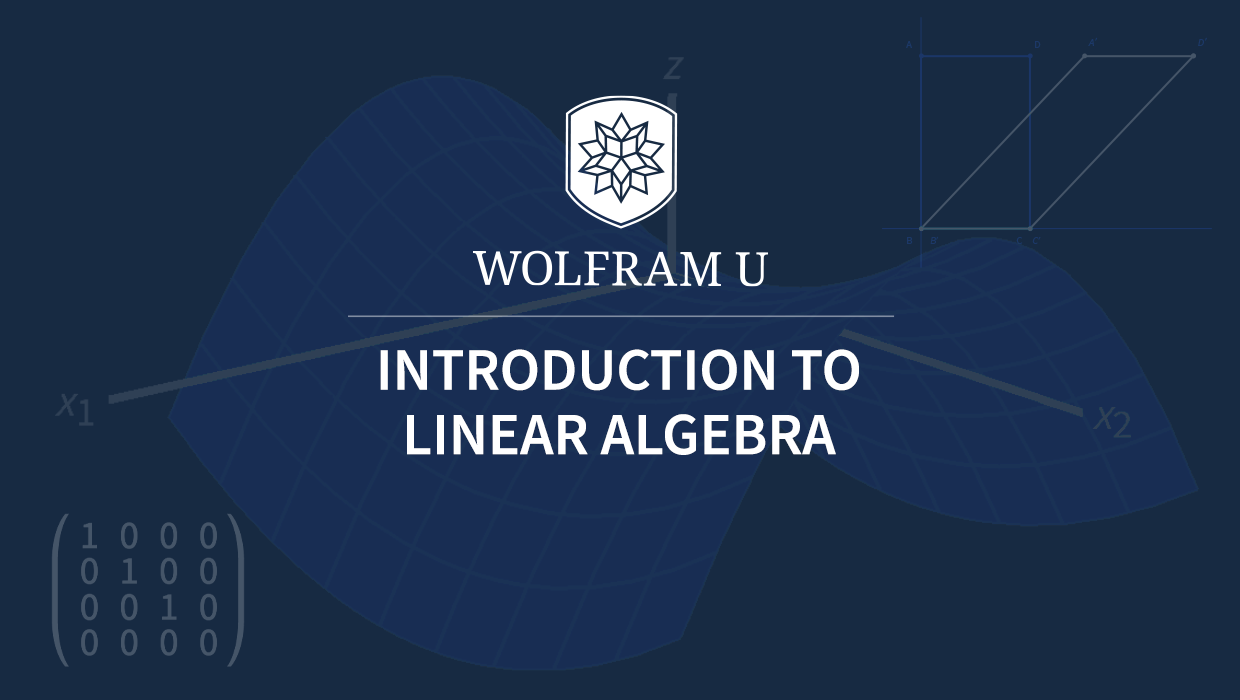Linear algebra is probably the easiest and the most useful branch of modern mathematics. Indeed, topics such as matrices and linear equations are often taught in middle or high school. On the other hand, concepts and techniques from linear algebra underlie cutting-edge disciplines such as data science and quantum computation. And in the field of numerical analysis, everything is linear algebra!

Today, I am proud to announce a free interactive course, Introduction to Linear Algebra, that will help students all over the world to master this wonderful subject. The course uses the powerful functions for matrix operations in the Wolfram Language and addresses questions such as “How long would it take to solve a system of 500 linear equations?” or “How does data compression work?”

I invite you to start exploring the interactive course by clicking anywhere in the following image before reading the rest of this blog post.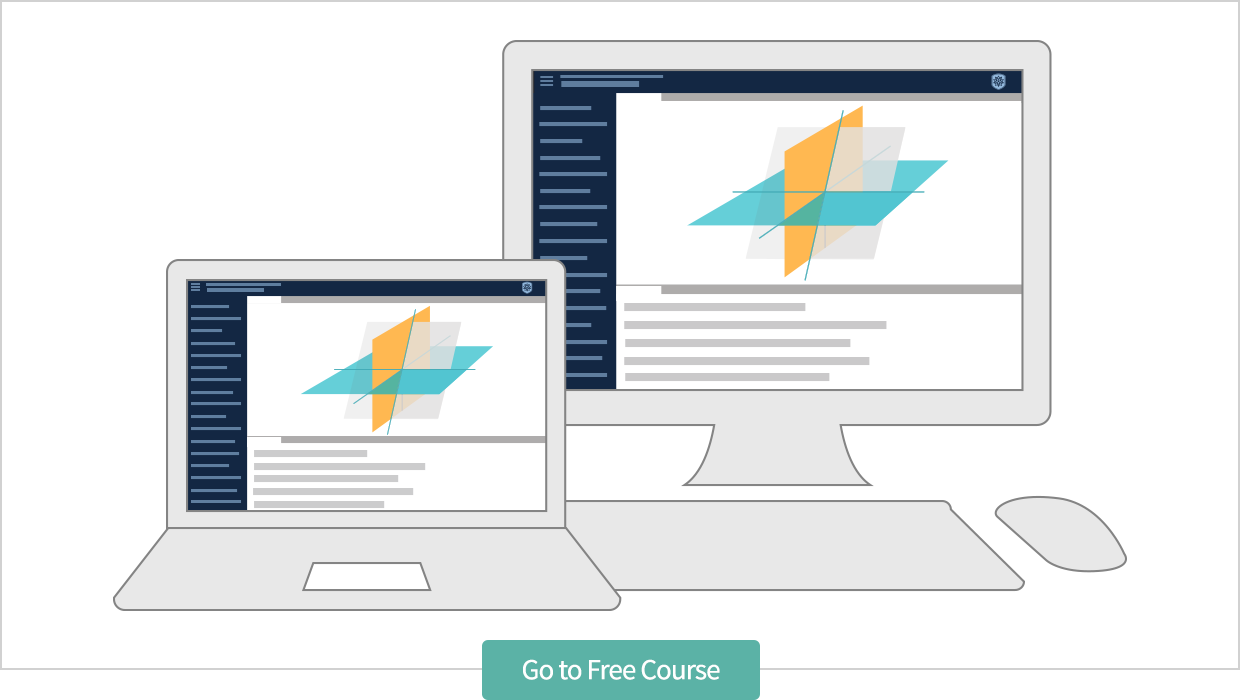## Motivation from History

The ancient Babylonians and Chinese knew how to solve simple systems of linear equations with two or three equations. However, the first systematic method for solving linear systems was given in 1750 by Gabriel Cramer, who formulated a rule for solving such systems using determinants. This was followed by the 1810 work of Carl Friedrich Gauss, who developed the technique known as Gaussian elimination for solving linear systems. Next, in 1850, James Joseph Sylvester introduced the notion of a matrix to represent arrays of numbers such as those formed by the coefficients of a linear system. A few years later, around 1855, Arthur Cayley published his work on the theory of matrices and operations on them. Finally, in 1888, Giuseppe Peano defined the notion of an abstract vector space, which plays a unifying role in modern linear algebra.

In keeping with the historical development, Introduction to Linear Algebra focuses on matrices and determinants, while vector spaces are discussed only when necessary during the course.

## Overview

Students taking this course will receive a thorough introduction to linear algebra including standard topics, such as linear systems, geometric transformations, matrix operations, determinants and eigenvalues. The course also includes a few advanced topics, such as the singular value decomposition, one of the most valuable concepts in applied linear algebra. Here is a sneak peek at some of the topics in the course (shown in the left-hand column):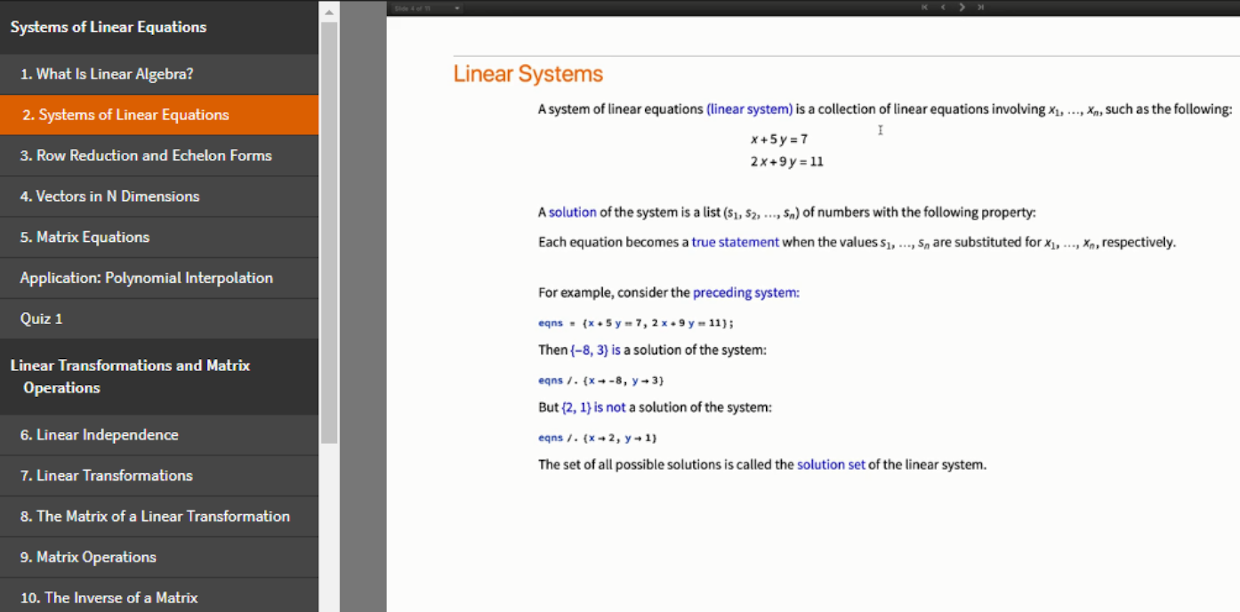I have worked hard to keep the course down to a reasonable length, and I expect that you will finish watching the 29 videos and also complete the five short quizzes in about five hours. (Hence the title of the blog post!)

Finally, I assume that the students are familiar with high-school algebra and basic trigonometry, but no calculus is required for this course.

The next few sections of the blog post will describe the different components of the course in detail.

## Lessons

The heart of the course is a set of 25 lessons, beginning with “What Is Linear Algebra?”. This introductory lesson includes a discussion of the different approaches to linear algebra, followed by a brief history of the subject and an outline of the course. Here is a short excerpt from the video for this lesson: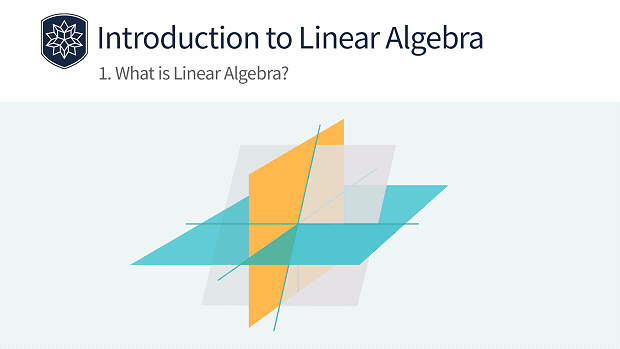Further lessons begin with an overview of the topic (for example, eigenvalues and eigenvectors), followed by a discussion of the key concepts interspersed with examples that illustrate the ideas using Wolfram Language functions for matrix computations.

The videos range from 7 to 12 minutes in length, and each video is accompanied by a transcript notebook displayed on the right-hand side of the screen. You can copy and paste Wolfram Language input directly from the transcript notebook to the embedded scratch notebook to try the examples for yourself.

## Exercises

Each lesson is accompanied by a set of five exercises to review the concepts covered during the lesson. Since this course is designed for independent study, a detailed solution is given for all exercises. The following shows an exercise from lesson 14 on applications of determinants: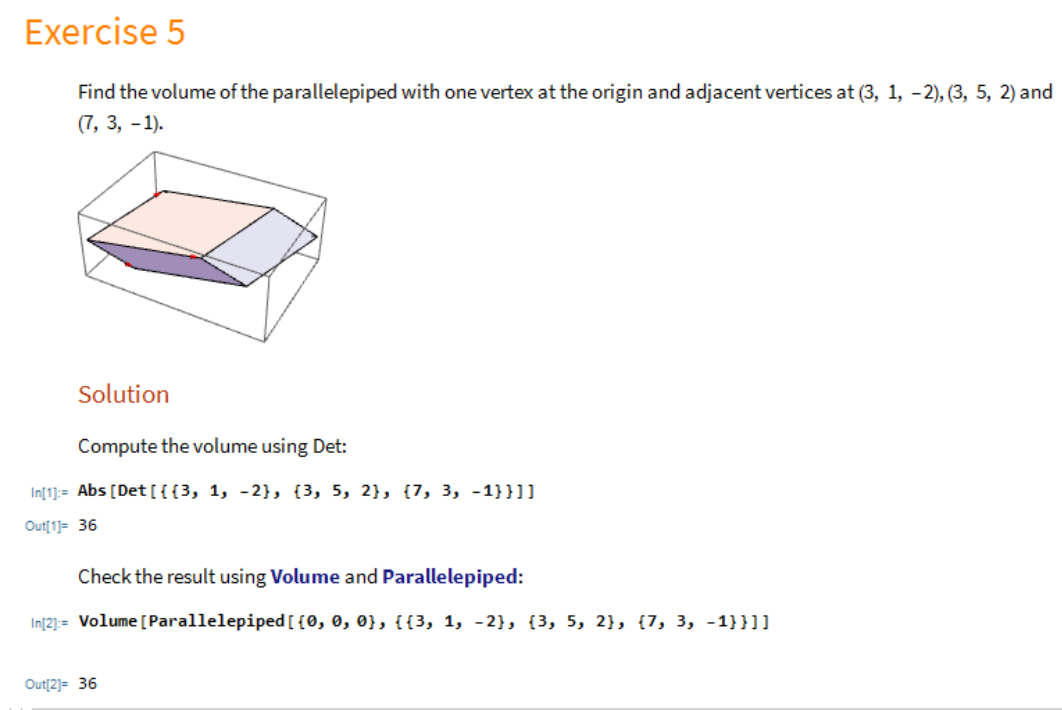The notebooks with the exercises are interactive, so students can try variations of each problem in the Wolfram Cloud. In particular, they are encouraged to change the entries of the matrices, vary the dimensions, etc. and experience the awesome power of the Wolfram Language for matrix computations.

## Application Sessions

Each section of the course includes an application session, which discusses a real-world application of the ideas developed in that section. There are four application sessions on polynomial interpolation, economic models, quantum entanglement and data compression, respectively. The following is a short excerpt from the video for data compression: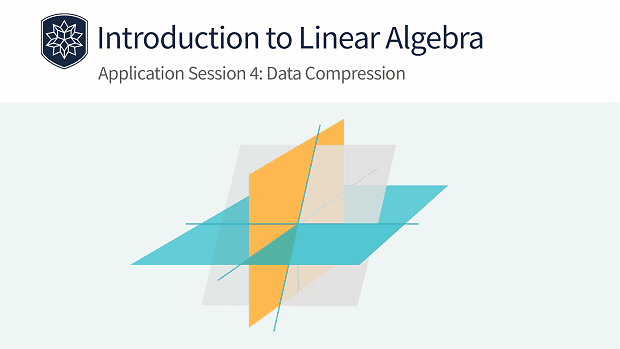These application sessions celebrate the great success of linear algebra techniques in engineering, computer science and other fields. I would like to thank Roger Germundsson, director of R&D at Wolfram, who suggested their inclusion in the course.

## Quizzes

Each section of the course ends with a short, multiple-choice quiz with five problems. The quiz problems are roughly at the same level as those discussed in the lessons, and a student who reviews the section carefully should have no difficulty in doing well on the quiz.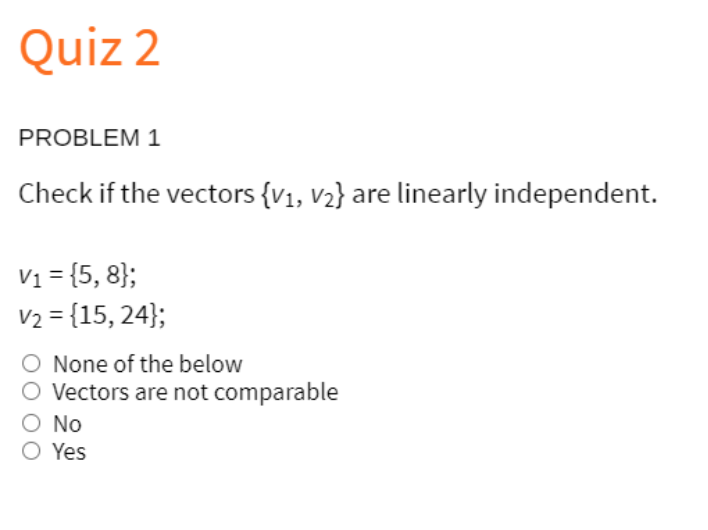Students will receive instant feedback about their responses to the quiz questions, and they are encouraged to try any method (including hand or computer calculations) to solve them.

## Course Certificate

I strongly encourage students to watch all the lessons and attempt the quizzes in the recommended sequence, since each topic in the course relies on earlier concepts and techniques. You can request a certificate of completion, pictured here, at the end of the course. A course certificate is achieved after watching all the lesson and application videos and passing all the quizzes. It represents proficiency in the fundamentals of linear algebra and will add value to your resume or social media profile.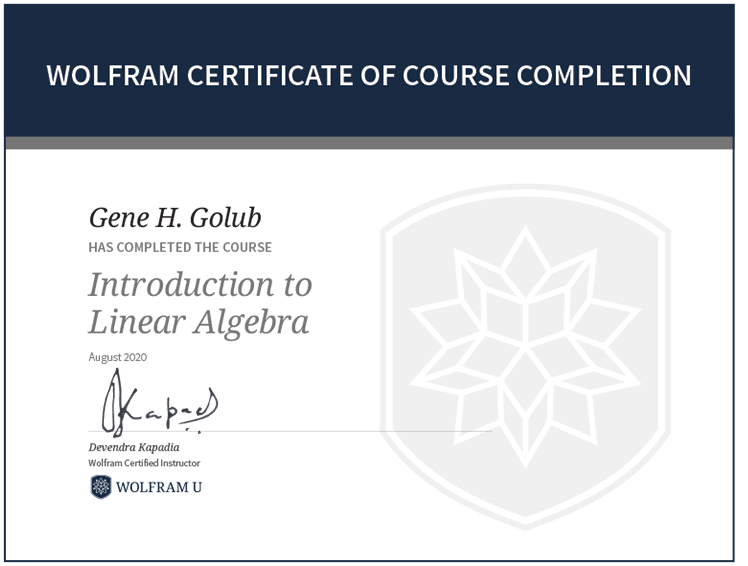There is also an optional final exam at the end of the course. You can receive an advanced level of certification by passing this exam.

## A Building Block for Success

A mastery of the fundamental concepts of linear algebra is crucial for students of data science, engineering and other fields. I hope that Introduction to Linear Algebra will help you to achieve such a mastery and will drive you toward success in your chosen field. I have enjoyed teaching the course and welcome any comments regarding the current course as well as suggestions for future courses.

## Acknowledgements

I would like to thank Vishaal Ganesan, Knarik Hovhannisyan, Veronica Mullen, Joyce Tracewell, Aram Manaselyan, Tim Shedelbower, Harry Calkins, Amruta Behera, Andy Hunt and Cassidy Hinkle for their dedicated work on various aspects (lessons, exercises, videos, etc.) of the course.

 Need help with calculus? Register for Wolfram U’s daily calculus study group.